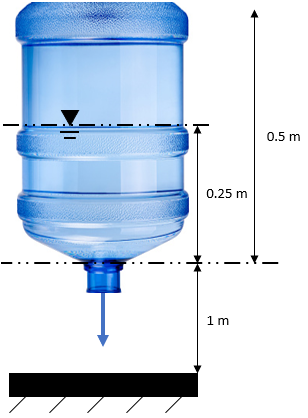## Jet Velocity

A 0.5 m tall water cooler jug is flipped upside down to freely flow water onto the ground through the jug's opening. If the jug was 1 m above the floor when flipped and losses are neglected, what is the jet velocity when the jug is half full of water?Hint
$$\frac{P_1}{\rho g}+z_1+\frac{v_{1}^{2}}{2g}=\frac{P_2}{\rho g}+z_2+\frac{v_{2}^{2}}{2g}+h_f$$$where $$h_f$$ is head loss, $$P_1$$ and $$P_2$$ are the pressures at sections 1 and 2, $$v_1$$ and $$v_2$$ are the average fluid velocities at the sections, $$z_1$$ and $$z_2$$ are the vertical distances from a datum to the sections (the potential energy), $$\rho$$ is the fluid density, and $$g$$ is gravity. Hint 2 In our situation, the following are negligible or cancel out: $$P_1=0$$ since the water jug is not internally pressurized → zero gauge $$v_1=0$$ since the tank is relatively large, meaning no noticeable movement at the water line $$P_2=0$$ since it is the pressure at the opening → atmospheric, which is zero gauge $$z_2=0$$ since there is no potential energy (height difference) at the exit point, if we're finding the speed at the exit point (the jug suspension is there to throw you off) $$h_f =0$$ since the problem statement defined head loss as negligible The energy equation for steady incompressible flow with no shaft device is $$\frac{P_1}{\rho g}+z_1+\frac{v_{1}^{2}}{2g}=\frac{P_2}{\rho g}+z_2+\frac{v_{2}^{2}}{2g}+h_f$$$
where $$h_f$$ is head loss (friction effect), $$P_1$$ and $$P_2$$ are the pressures at sections 1 and 2, $$v_1$$ and $$v_2$$ are the average fluid velocities at the sections, $$z_1$$ and $$z_2$$ are the vertical distances from a datum to the sections (the potential energy), $$\rho$$ is the fluid density, and $$g$$ is gravity.

In our situation, the following are negligible or cancel out:
$$P_1=0$$ since the water jug is not internally pressurized → zero gauge
$$v_1=0$$ since the tank is relatively large, meaning no noticeable movement at the water line
$$P_2=0$$ since it is the pressure at the opening → atmospheric, which is zero gauge
$$z_2=0$$ since there is no potential energy (height difference) at the exit point, if we're finding the speed at the exit point (the jug suspension is there to throw you off)
$$h_f =0$$ since the problem statement defined head loss as negligible
Thus,
$$z_1=\frac{v_{2}^{2}}{2g}$$$$$v_{2}^{2}=z_1(2g)\rightarrow v_2=\sqrt{z_1(2g)}$$$
$$v_2=\sqrt{(0.25m)(2)(9.8m/s^2)}=\sqrt{4.9m^2/s^2}=2.2\:m/s$$\$
2.2 m/s